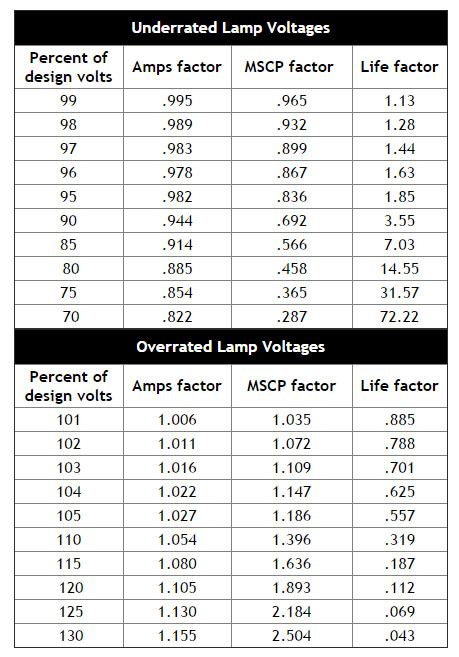908.508.9100 sales@lumitron.com

The three operating factors that most contribute to shortened lamp life are:

• Overheating (lamp envelope temperature should not exceed 100 degrees C);
• Power fluctuations; and
• Mechanical shock and vibration (particularly when the lamp is on).

Keep-Alive current: Because the resistance of tungsten filaments is far greater when the filament is cold, supplying a small current (as little as one percent of operating voltage) to the lamp when switched off will keep the filament warm and buffer it against inrush current when switched on. This greatly extends lamp life.

Lamp Rerating:

Rerating a standard lamp (adjusting the voltage above or below the stated design figure) can frequently produce the desired relationship of current, life, and brightness, avoiding the expense of a custom design. Changing the voltage affects other characteristics according to the formulas and the chart below.

Rerating formulas:

Candlepower is directly proportional to the 3.5 power of the ratio of the applied voltage, and can be increased at the expense of lamp life.

Current consumption is approximately proportional to the 0.55 power of the ratio of applied voltage versus the rated voltage.

Other rerating formulas in our print catalog can be used to calculate the effects of lamp rerating. The interrelationship of voltage, current, MSCP, and life for a particular lamp can be found on the charts below. Simply multiply the rated figures by the factors to find the effects of rerating at specific percentages of design volt.

Example: Lumitron P/N L-715-AS15 is a 5-volt lamp with .150 MSCP, 115mA current draw, and 40,000 hour life. Operating the lamp at 6 volts, or 120 percent of design volts, yields the following characteristics:

MSCP: Multiplying the 5-volt MSCP figure of .150 by 1.893 (from the chart) yields an MSCP of .284 when the lamp is operated at 6 volts.

mA: Multiplying the 5-volt mA figure of .115 by 1.105 (from the chart) yields a current draw of .127 mA when the lamp is operated at 6 volts.

Life: Multiplying the 5-volt life figure of 40,000 hours by .112 (from the chart) yields a life expectancy of 4,480 hours when the lamp is operated at 6 volts.